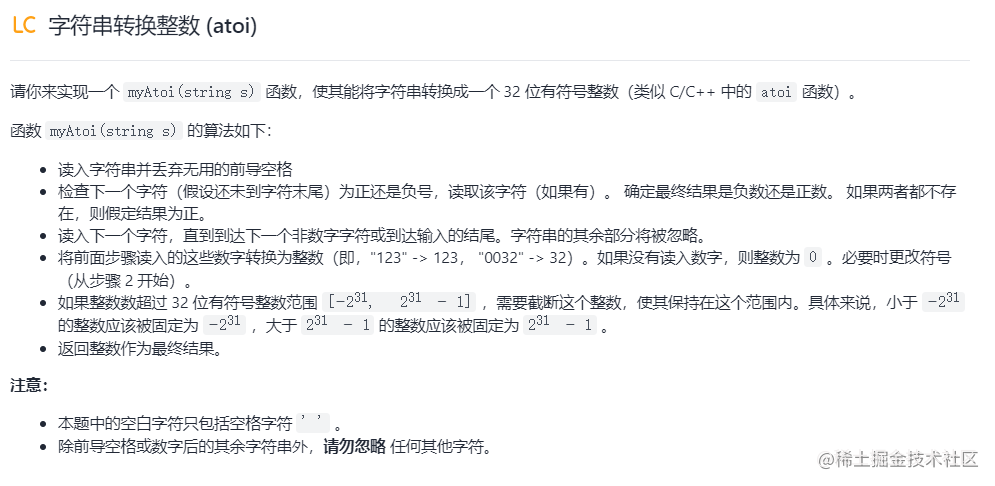# LeetCode《初級演算法》字串之字串轉換整數 -- JavaScript

## 題目## 題解

### 1、按照題意一步一步來

```js /* * @param {string} s * @return {number} / var myAtoi = function(s) {

``````let index = 0,
isMinus = false,
integer = 0;

const max_abs = Math.pow(2,31) - 1,
min_abs = Math.pow(2,31);

while(s[index] === ' '){
index++;
}

if(s[index] === '-'){
index++;
isMinus = true;
}else if(s[index] === '+'){
index++;
}

while(s[index] === '0') {
index++;
}

while(s[index] <= '9' && s[index] >= '0') {

integer = integer * 10 + Number(s[index]);
if( integer > max_abs && false === isMinus) {
return max_abs;
}
if( integer > min_abs && true === isMinus) {
return 0 - min_abs;
}
index++;

}

if(integer !== 0 && isMinus) {
return -integer;
}
return integer;
``````

};

```

### 2、使用 JS Number物件給的 parseInt 方法

```js /* * @param {string} s * @return {number} / var myAtoi = function(s) {

``````let n = parseInt(s);
const max = Math.pow(2,31) - 1,
min = 0 - Math.pow(2,31);

if(n.toString() === 'NaN') {
return 0;
}else if( n < min) {
return min;
}else if( n > max ) {
return max;
}

return n;
``````

}; ```

https://juejin.cn/post/7006692002125316103